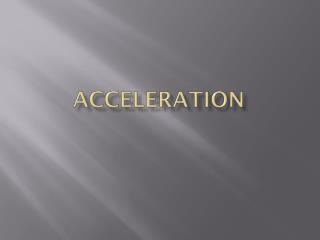DownloadDownload PresentationACCELERATION

# ACCELERATION

Télécharger la présentation## ACCELERATION

- - - - - - - - - - - - - - - - - - - - - - - - - - - E N D - - - - - - - - - - - - - - - - - - - - - - - - - - -
##### Presentation Transcript

1. ACCELERATION

2. Acceleration, Speed and Velocity -Acceleration = rate of change of velocity. -A change in velocity can be either a change in how fast something is moving, or a change in the direction it is moving. -Acceleration occurs when an object changes its speed, it's direction, or both.

3. If the acceleration is in the same direction as the velocity, the speed increases and the acceleration is positive. Speeding Up and Slowing Down

4. If the speed decreases, the acceleration is in the opposite direction from the velocity, and the acceleration is negative. Speeding Up and Slowing Down

5. Changing Direction The speed of the horses in this carousel is constant, but the horses are accelerating because their direction is changing constantly.

6. Acceleration Calculating Acceleration To calculate the acceleration of an object, the change in velocity is divided by the length of time interval over which the change occurred.

7. Acceleration Calculating Acceleration So, the formula for acceleration is:

8. Calculating Positive Acceleration • -How is the acceleration for an object that is speeding up different from that of an object that is slowing down? • -Positive acceleration = “speeding up” • -Negative acceleration = “slowing down”- -Suppose a jet airliner starts at rest at the end of a runway and reaches a speed of 80 m/s in 20 s.

9. Acceleration Calculating Positive Acceleration Its acceleration can be calculated as follows:

10. Acceleration Calculating Positive Acceleration The airliner is speeding up, so the final speed is greater than the initial speed and the acceleration is positive.

11. Acceleration Now imagine that a skateboarder is moving in a straight line at a constant speed of 3 m/s and comes to a stop in 2 s. Calculating Negative Acceleration The final speed is zero and the initial speed was 3 m/s.

12. Acceleration Calculating Negative Acceleration The skateboarder's acceleration is calculated as follows: a=(vf-vi)/t a=(0-3)/2 a= -1.5m/s2

13. Acceleration The skateboarder is slowing down, so the final speed is less than the initial speed and the acceleration is negative. Calculating Negative Acceleration

14. Graphing Motion • The slope of the d vs. t graph is the velocity. • The slope of the v vs. t graph is the acceleration. • The area of the v vs. t graph is the change in position. • The area of the a vs. t graph is the change in velocity. • http://jersey.uoregon.edu/vlab/block/Block.html

15. Position with Average Acceleration • What if we want to combine our velocity and acceleration equations? • If the initial time is ti=0 then we can use the following equation to calculate position with average acceleration • df = di + vi(tf) + ½a(tf)2

16. Velocity with Constant Acceleration • What if we don’t have time? • The square of the final velocity equals the sum of the square of the initial velocity and twice the product of the acceleration and the displacement since the initial time • vf2=vi2 + 2a(df-di)

17. Equations v = (df-di)/(tf-ti)  a = (vf-vi)/(tf-ti) df = di + vi(tf) + ½a(tf)2 vf2 = vi2+ 2a(df-di)